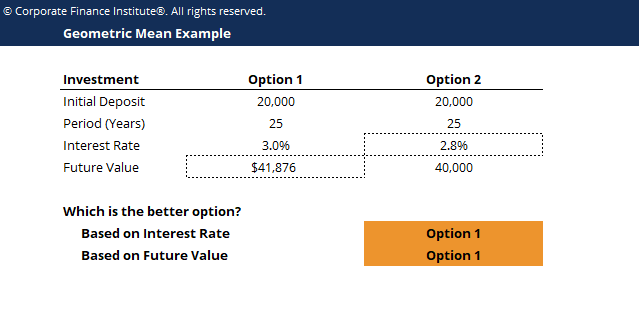# Geometric Mean Template

## Geometric Mean Template

This geometric mean template helps you compare investment options by calculating the final value of investments using geometric mean.

Below is a preview of the geometric mean template:### Geometric Mean Template

The geometric mean is the average growth of an investment computed by multiplying n variables and then taking the n square root.  In other words, it is the average return of an investment over time, a metric used to evaluate the performance of an investment portfolio.

### Why use Geometric Mean?

The arithmetic mean is the calculated average of the middle value of a data series; it is accurate to take an average of independent data, but weakness exists in continuous data series calculation. The geometric mean is used to tackle continuous data series which the arithmetic mean is unable to calculate.

### Geometric Mean Formula for Investments

Geometric Mean = [Product of (1 + Rn)] ^ (1/n) -1

Where Rn = growth rate for year N

### More Free Templates

For more resources, check out our business templates library to download numerous free Excel modeling, PowerPoint presentation and Word document templates.

• Excel Modeling Templates
• PowerPoint Presentation Templates
• Transaction Document Templates

### Financial Analyst Certification

Become a certified Financial Modeling and Valuation Analyst (FMVA)® by completing CFI’s online financial modeling classes and training program!